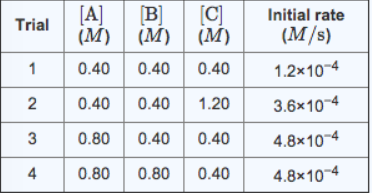# Problem: For the reaction-A + B + C → D + Ethe initial raction rate was measured for various initial concentrations of reactants. The follwoing data were collected:a. What is the reaction order with respect to A?b. What is the reaction order with respect to B?c. What is the reaction order with respect to C?d. What is the value of the rate constant k for this reaction?e. Given the data calculated in Parts A, B, C, and D, determine the initial rate for a reaction that starts with 0.55M of reagent A and 0.70M of reagents B and C?

###### FREE Expert Solution

We are being asked to determine the following for the reaction

A + B + C → D + E

We will do the following steps:

Step 1: Determine the reaction order with respect to A

Step 2: Determine the reaction order with respect to B

Step 3: Determine the reaction order with respect to C

Step 4: Determine the rate law

Step 5: Determine the rate constant k for the reaction

Step 6: Determine the initial rate given the concentrations of the reactants

88% (74 ratings)###### Problem Details

For the reaction-

A + B + C → D + E

the initial raction rate was measured for various initial concentrations of reactants. The follwoing data were collected:

a. What is the reaction order with respect to A?

b. What is the reaction order with respect to B?

c. What is the reaction order with respect to C?

d. What is the value of the rate constant k for this reaction?

e. Given the data calculated in Parts A, B, C, and D, determine the initial rate for a reaction that starts with 0.55M of reagent A and 0.70M of reagents B and C?